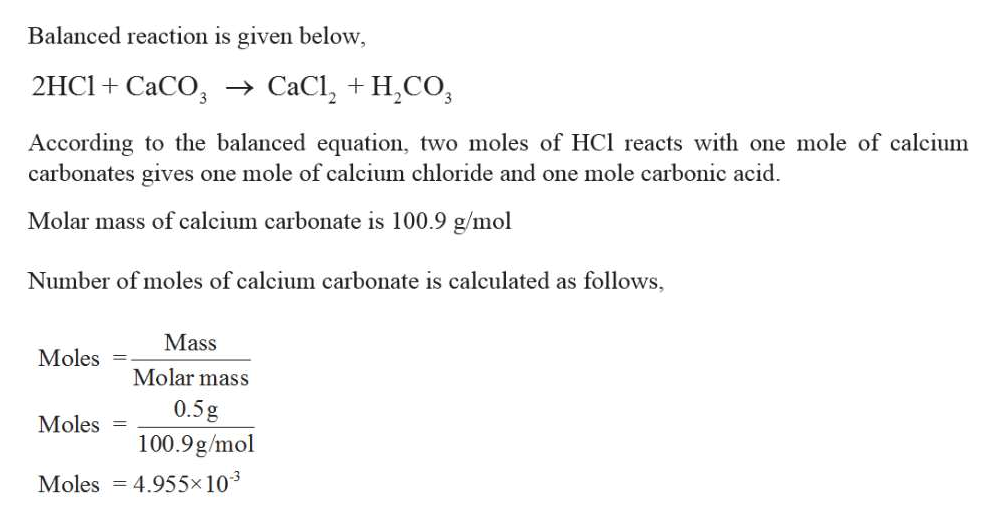# Stomach acid is approximately 0.10 M HCl. How many mL of stomach acid can be neutralized by one regular antacid tablet that contains 500 mg of solid CaCO3 (100.9 g/mol) ?

Question
2 views

Stomach acid is approximately 0.10 M HCl. How many mL of stomach acid can be neutralized by one regular antacid tablet that contains 500 mg of solid CaCO3 (100.9 g/mol) ?

check_circle

Step 1

Given,

0.10 M HCl.

500 mg of soli...help_outlineImage TranscriptioncloseBalanced reaction is given below, 2HC1 CaCO -> CaCl, + H,CO According to the balanced equation, two moles of HCl reacts with one mole of calcium carbonates gives one mole of calcium chloride and one mole carbonic acid Molar mass of calcium carbonate is 100.9 g/mol Number of moles of calcium carbonate is calculated as follows, Mass Moles Molar mass 0.5g Moles 100.9g/mol Moles 4.955x 103 fullscreen

### Want to see the full answer?

See Solution

#### Want to see this answer and more?

Solutions are written by subject experts who are available 24/7. Questions are typically answered within 1 hour.*

See Solution
*Response times may vary by subject and question.
Tagged in

### Chemistry